Questions from the forum
 Q: How can I attach a "real", working headlight to one of my models? A: Here's an example; use the cursor keys to move the model around.   function my_headlight() {        set(my, PASSABLE);        vec_set(d3d_spotlightcone, vector(25, 80, 4)); // play with these values        vec_set(my.blue, vector(255, 255, 255)); // the headlight will generate white light        my.lightrange = 500; // on a range of up to 500 quants        my.flags2 |= SPOTLIGHT;        while (1)        {                // play with the numerical values, they set the position of the headlight (x, y, z)                vec_set (my.x, vector (10, 0, 50));                vec_rotate (my.x, you.pan);                vec_add (my.x, you.x);                my.pan = you.pan;                wait (1);        } }   action my_model() // simple entity movement code using the cursor keys {        ent_create("headlight.mdl", nullvector, my_headlight); // create a tiny headlight model        while (1)        {                c_move (my, vector(10 * (key_cuu - key_cud) * time_step, 0, 0), nullvector, GLIDE | IGNORE_PASSABLE);                my.pan += 6 * (key_cul - key_cur) * time_step;                wait (1);        } }     Q: I would like to have a task strip below the screen that rises when the mouse is placed at the bottom of the screen and includes clickable pictures. A: Here's an example.   var strip_ready = 0;   BMAP* pointer_tga = "pointer.tga"; BMAP* mypanel_pcx = "mypanel.pcx"; // this is your "task strip" bitmap BMAP* picture1on_pcx = "picture1on.pcx"; BMAP* picture1off_pcx = "picture1off.pcx"; BMAP* picture2on_pcx = "picture2on.pcx"; BMAP* picture2off_pcx = "picture2off.pcx";   function test1(); function test2();   PANEL* taskstrip_pan = {        bmap = mypanel_pcx;        pos_x = 0;        pos_y = 598; // this will work fine for a 800x600 pixels resolution (subtract 2 from the y resolution value = 600)        button(1, 1, picture1on_pcx, picture1off_pcx, picture1on_pcx, test1, NULL, NULL);        button(41, 1, picture2on_pcx, picture2off_pcx, picture2on_pcx, test2, NULL, NULL);        flags = VISIBLE; }   function mouse_startup() {         mouse_mode = 2;        mouse_map = pointer_tga;        while (1)        {                 vec_set(mouse_pos, mouse_cursor);                wait(1);        } }   function slide_panel_startup() {        while (1)        {                if ((mouse_pos.y > 598) && (!strip_ready)) // play with this value, it depends on the game's screen resolution                {                        while (taskstrip_pan.pos_y > 568) // slide until the entire panel is visible (it has a height 32 pixels)                        {                                taskstrip_pan.pos_y -= 10 * time_step; // 10 gives the sliding speed                                wait (1);                                                       }                        taskstrip_pan.pos_y = 568;                        strip_ready = 1; // allow the panel to be visible even if the mouse pointer is moved a bit upwards                }                if (mouse_pos.y < 568) // the player has moved the mouse away from the panel?                {                        while (taskstrip_pan.pos_y < 598) // hide the task strip; allow only 2 pixels from it to be visible                        {                                taskstrip_pan.pos_y += 10 * time_step; // 10 gives the sliding speed                                wait (1);                                                       }                        taskstrip_pan.pos_y = 598;                        strip_ready = 0; // the player can't control the pictures on the task strip panel anymore                }                wait (1);        } }   function test1() {        beep(); }   function test2() {        beep(); beep(); }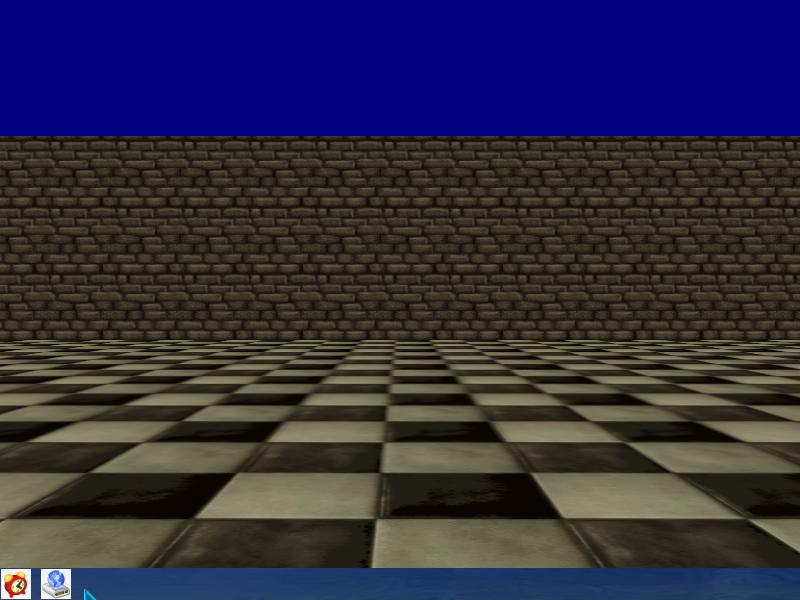Q: I have a function that shows a panel and activates the cursor. If I trigger it with event_impact, the cursor freezes. If I call it with a keystroke, the cursor works. What is wrong with my code?   function barrel_event() {        if (event_type == EVENT_IMPACT)        {                snd_play(gong, 40, 0);                show_challenge();                ent_remove(me); // disappear when hit        } }   A: Your barrel_event function is called several times on impact, while a keystroke only triggers the function once. Use "proc_kill (4);" to terminate all the other instances of barrel_event or set the event function to NULL after its first call, just like this:   function barrel_event() {        my.event = NULL; // the function won't be called again after the first impact        if (event_type == EVENT_IMPACT)        {                snd_play(gong, 40, 0);                show_challenge();                ent_remove(me); // disappear when hit        } }     Q: I have an fps gun which animates using the left mouse button; however, if I release the mouse button, the animation will stop without completing the cycle. Can anyone help? A: Use this example as a base for your code.   ENTITY* myweapon_ent = {        type = "myweapon.mdl"; // your weapon model        pan = 0; // weapon's angle        x = 55; // 55 quants ahead of the view, play with this value        y = -20; // 20 quants towards the right side of the screen, play with this value        z = -20; // 20 quants below, play with this value        pan = 2; // weapon's pan angle (set its tilt, roll, etc if you want to)        flags2 = VISIBLE;                }   function animate_startup() {        var anim_percentage = 0;        while (1)        {                while (!mouse_left) {wait (1);}                while (anim_percentage < 100)                {                        anim_percentage += 6 * time_step;                        ent_animate(myweapon_ent, "shoot", anim_percentage, NULL); // play a one-shot animation                        wait (1);                }                anim_percentage = 0;                while (mouse_left) {wait (1);}        } }     Q: My code uses the c_move instruction; however, the model penetrates the wall partially, just like in the picture below. What is wrong?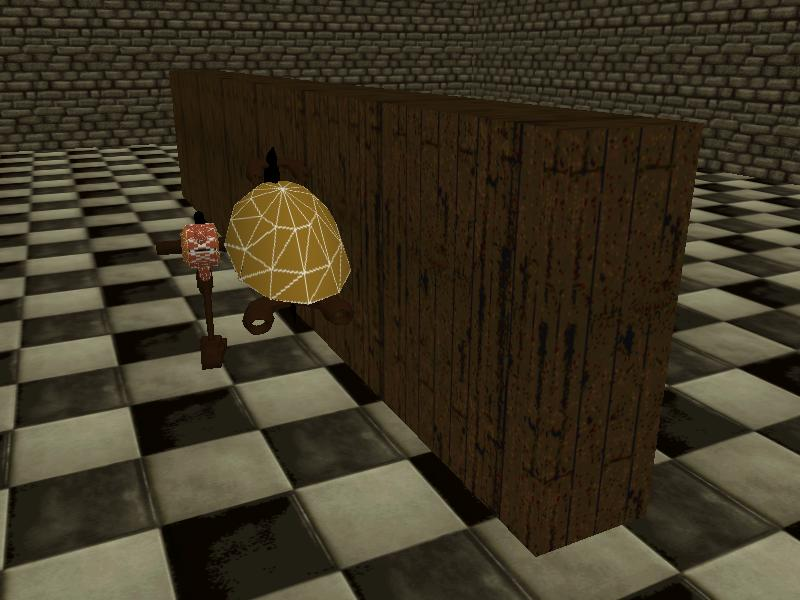A: Press F11 twice; you will see the actual hull size and shape of your entity; it's a box that works very fast when it comes to collision checks.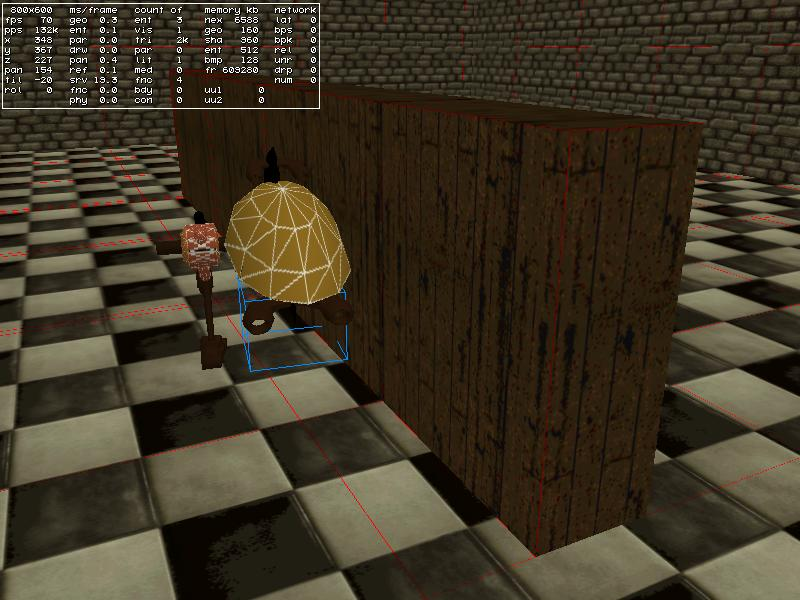The solution is simple: add a single "c_setminmax(my);" line of code to your movement code.   action my_model( ) // simple movement code example {        c_setminmax(my); // add this line to your movement code        while (1)        {                c_move (my, vector(10 * (key_w - key_s) * time_step, 0, 0), nullvector, GLIDE); // move using the WS keys                my.pan += 6 * (key_a - key_d) * time_step; // rotate using the AD keys                wait (1);        } }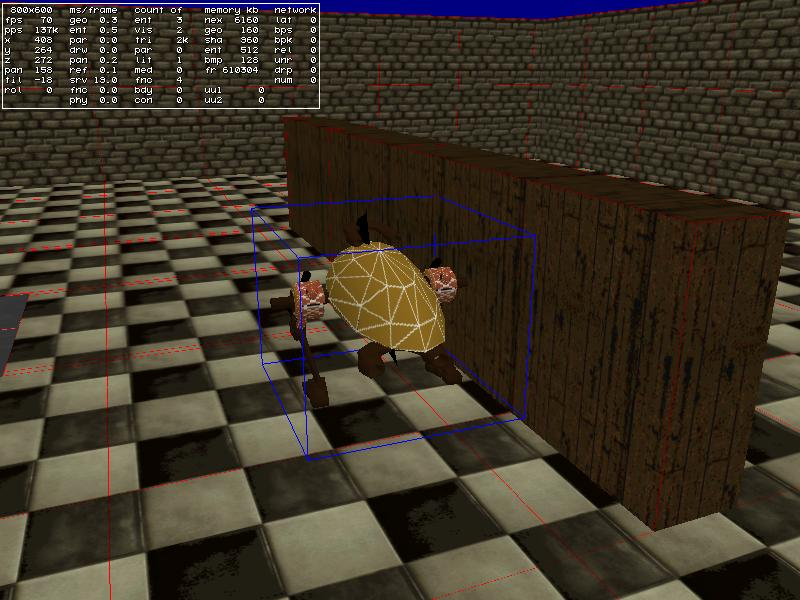Q: How can I use the code snippets from Aum's faq? Do I save them as separate files, and then copy the files to my code folder? Or is there a step that I am missing? A: Here's a step by step explanation:   1) Create a new lite-C file using the script editor (File -> New). 2) Copy the code from the magazine and then paste it inside the newly created file. 3) Save the file inside your game folder. 4) Include the .c file in your project using a line of code that looks like this:   #include "mynewscript.c"; // use your own script name here   5) Create or copy all the resources that are needed by the snippet (graphics, sounds, models, etc) to your game folder. 6) Attach the actions (if any) to your models using Wed. That's it!     Q: I can't get a flash sprite to animate at the gun muzzle. Can anyone help? A: Use this snippet.   VECTOR trace_coords;   STRING* hithole_tga = "hithole.tga"; // that's your hit hole bitmap STRING* target_mdl = "target.mdl"; // weapon target model SOUND* bullet_wav = "bullet.wav"; // bullet sound   function fire_bullets(); // creates the bullets function show_target(); // displays the (red) target model function display_hithole(); // shows the hit hole bitmap   ENTITY* gun;   function attach_muzzle() {        set (my, PASSABLE | BRIGHT);        my.scale_x = 0.04; // play with the scale of the muzzle sprite        my.scale_y = my.scale_x;        my.frame = 1;        while (my.frame < 20) // using a sprite muzzle with 20 animation frames        {                my.frame += 5 * time_step;                vec_set (my.x, vector (29, 1, 3)); // muzzle offset in relation to the gun                vec_rotate (my.x, gun.pan);                vec_add (my.x, gun.x);                my.pan = gun.pan;                my.tilt = gun.tilt;                wait (1);        } }   function attach_weapon() {        gun = my; // I'm the gun        set(my, PASSABLE);        while (1)        {                vec_set (my.x, vector (10, -10, 40)); // gun offset in relation to the player                vec_rotate (my.x, you.pan);                vec_add (my.x, you.x);                my.pan = you.pan;                my.tilt = camera.tilt;                wait (1);        } }   action my_player() // attach this action to your player {        ent_create("weapon1.mdl", nullvector, attach_weapon);        var movement_speed = 10; // movement speed        VECTOR temp;        player = my; // I'm the player        set (my, INVISIBLE); // 1st person player        while (1)        {                player.pan -= 7 * mouse_force.x * time_step;                camera.x = player.x;                camera.y = player.y;                camera.z = player.z + 50 + 1.1 * sin(my.skill44); // play with 50 and 1.1                camera.pan = player.pan;                camera.tilt += 5 * mouse_force.y * time_step;                vec_set (temp.x, my.x); // trace 10,000 quants below the player                temp.z -= 10000;                temp.z = -c_trace (my.x, temp.x, IGNORE_ME | IGNORE_PASSABLE | USE_BOX);                temp.x = movement_speed * (key_w - key_s) * time_step;                temp.y = movement_speed * (key_a - key_d) * 0.6 * time_step;                c_move (my, temp.x, nullvector, IGNORE_PASSABLE | GLIDE);                wait (1);        } }   function weapon_startup() {        on_mouse_left = fire_bullets; // call this function when the left mouse button is pressed        proc_mode = PROC_LATE; // run this function at the end of the function scheduler list (eliminates jerkiness)        while (1)        {                vec_set(trace_coords.x, vector(10000, 0, 0)); // the weapon has a firing range of up to 10,000 quants                vec_rotate(trace_coords.x, camera.pan);                vec_add(trace_coords.x, camera.x);                if (c_trace(camera.x, trace_coords.x, IGNORE_ME | IGNORE_PASSABLE) > 0) // hit something?                {                        ent_create (target_mdl, target.x, show_target); // then show the target model                }                wait (1);        } }   function show_target() {        set (my, PASSABLE); // the target model is passable        my.ambient = 100; // and should look bright enough        my.scale_x = minv (6, vec_dist (my.x, camera.x) / 500); // play with 6 and with 500        my.scale_y = my.scale_x;        my.scale_z = my.scale_x;        wait (1);        ent_remove (my); }   function fire_bullets() {        while (mouse_left)        {                c_trace(camera.x, trace_coords.x, IGNORE_ME | IGNORE_PASSABLE | ACTIVATE_SHOOT);                if (!you) // hit a wall?                {                        ent_create (hithole_tga, target.x, display_hithole); // then create a bullet hit hole                }                snd_play (bullet_wav, 100, 0); // play the bullet sound at a volume of 100                ent_create("muzzle+20.tga", nullvector, attach_muzzle);                wait (-0.16); // fire 6 bullets per second (6 * 0.16 ~= 1 second)        } }   function display_hithole() {        vec_to_angle (my.pan, normal); // orient the hit hole sprite correctly        vec_add(my.x, normal.x); // move the sprite a bit away from the wall        set (my, PASSABLE); // the hit hole bitmap is passable        set (my, TRANSLUCENT); // and transparent        my.ambient = 50;        my.roll = random(360); // and has a random roll angle        my.scale_x = 0.5; // we scale it down        my.scale_y = my.scale_x; // on the x and y axis        wait (-20); // show the hit hole bitmap for 20 seconds        ent_remove (my); // and then remove it }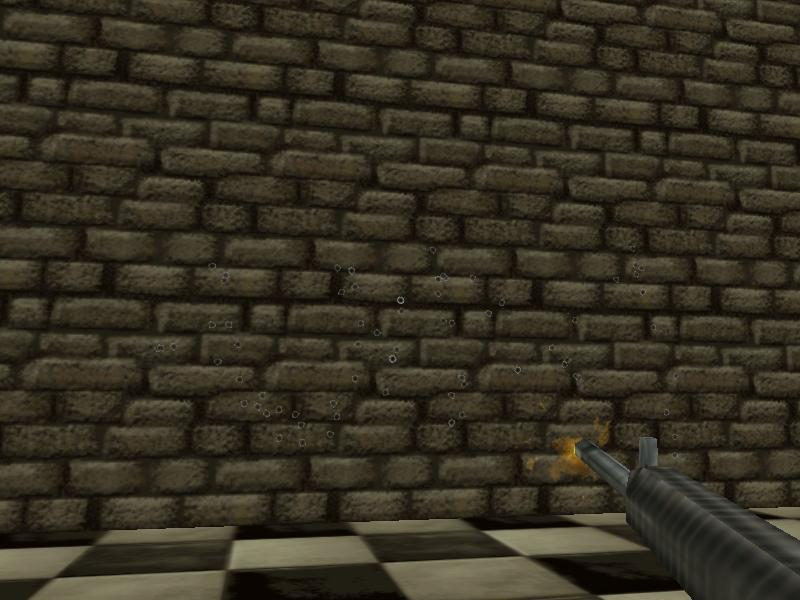Q: I would like to be able to blow up a barrel with flames and smoke. A: Here's an example.   BMAP* fire_tga = "fire.tga"; BMAP* smoke_tga = "smoke.tga";   function fade_fire(PARTICLE *p) {        p.alpha -= 4 * time_step; // fade out the fire particles        if (p.alpha < 0)                p.lifespan = 0; }   function fade_smoke(PARTICLE *p) {        p.alpha -= 0.5 * time_step; // fade out the fire particles        if (p.alpha < 0)                p.lifespan = 0; }   function fire_effect(PARTICLE *p) {        set (my, PASSABLE);        p->vel_x = 5 - random(10);        p->vel_y = 5 - random(10);        p->vel_z = 10 - random(20);        p.alpha = 50 + random(50);        p.bmap = fire_tga;        p.size = 15; // gives the size of the flame particles        p.flags |= (BRIGHT | MOVE);        p.event = fade_fire; }   function smoke_effect(PARTICLE *p) {        set (my, PASSABLE);        p->vel_x = 1 - random(2);        p->vel_y = 1 - random(2);        p->vel_z = 1 + random(2);        p.alpha = 10 + random(20);        p.bmap = smoke_tga;        p.size = 25; // gives the size of the smoke particles        p.flags |= MOVE;        p.event = fade_smoke; }   function explo_sprite() {        set(my, BRIGHT);        my.alpha = 100;        my.scale_x = 3; // this value gives the scale of the explosion sprite        my.scale_y = my.scale_x;        my.frame = 1;        while (my.frame < 5)        {                my.frame += 0.5 * time_step;                wait (1);        }        while (my.alpha > 0)        {                my.alpha -= 3 * time_step;        }        ent_remove (my); }   function got_shot() {        my.event = NULL; // don't react to other events from now on        set(my, PASSABLE); // the barrel is now passable        ent_create("explo+5.tga", my.x, explo_sprite);        effect(fire_effect, 1000, my.x, nullvector);        effect(smoke_effect, 100, my.x, nullvector);        my.alpha = 100;        set(my, TRANSLUCENT);        while (my.alpha > 0)        {                my.alpha -= 5 * time_step;                wait (1);        }        ent_remove(my); }   action explo_barrel() // attach this action to your barrels {        // make the barrel entity sensitive to c_trace and impact with other entities        my.emask |= (ENABLE_SHOOT | ENABLE_IMPACT | ENABLE_ENTITY);        my.event = got_shot; }     Q: I have a car and I would like to blow it up with smoke, flames and have it roll over on its side at the same time. A: Here you go.   BMAP* fire_tga = "fire.tga"; BMAP* smoke_tga = "smoke.tga";   function fade_fire(PARTICLE *p) {        p.alpha -= 4 * time_step; // fade out the fire particles        if (p.alpha < 0)                p.lifespan = 0; }   function fade_smoke(PARTICLE *p) {        p.alpha -= 1 * time_step; // fade out the fire particles        if (p.alpha < 0)                p.lifespan = 0; }   function fire_effect(PARTICLE *p) {        set (my, PASSABLE);        p->vel_x = 4 - random(8);        p->vel_y = 4 - random(8);        p->vel_z = 2 + random(5);        p.alpha = 20 + random(30);        p.bmap = fire_tga;        p.size = 25; // gives the size of the flame particles        p.flags |= (BRIGHT | MOVE);        p.event = fade_fire; }   function smoke_effect(PARTICLE *p) {        set (my, PASSABLE);        p->vel_x = 3 - random(6);        p->vel_y = 3 - random(6);        p->vel_z = 3 + random(5);        p.alpha = 5 + random(10);        p.bmap = smoke_tga;        p.size = 50; // gives the size of the smoke particles        p.flags |= MOVE;        p.event = fade_smoke; }   function explo_sprite() {        set(my, BRIGHT);        my.alpha = 100;        my.scale_x = 3; // this value gives the scale of the explosion sprite        my.scale_y = my.scale_x;        my.frame = 1;        while (my.frame < 5)        {                my.frame += 0.5 * time_step;                wait (1);        }        while (my.alpha > 0)        {                my.alpha -= 3 * time_step;        }        ent_remove (my); }   function car_hit() {        my.event = NULL; // don't react to other events from now on        ent_create("explo+5.tga", my.x, explo_sprite);        wait (-0.1);        my.skill1 = my.z; // store the initial height of the car        while (my.z < (my.skill1 + 500)) // allow the car to jump up to 500 quants        {                my.z += 50 * time_step;                if (my.roll < 270)                        my.roll += 10 * time_step;                wait (1);        }        my.skill10 = 0;        while (my.skill10 < 0.5) // keep the car at its maximum height for 0.5 seconds (for increased realism)        {                my.skill10 += time_step / 16;                if (my.roll < 270)                        my.roll += 10 * time_step;                wait (1);        }        while (my.z > (my.skill1 + 10)) // allow the car to return to the ground now, 10 = experimental value        {                my.z -= 40 * time_step;                if (my.roll < 270)                        my.roll += 10 * time_step;                wait (1);                        }        while (my.roll < 270) // make sure that the car has rotated for a full 270 degrees angle        {                my.roll += 10 * time_step;                wait (1);        }        while (1)        {                effect(fire_effect, 200, my.x, nullvector);                effect(smoke_effect, 50, my.x, nullvector);                wait (1);        } }   action explo_car() // attach this action to your car {        // make the car entity sensitive to c_trace and impact with other entities        my.emask |= (ENABLE_SHOOT | ENABLE_IMPACT | ENABLE_ENTITY);        my.event = car_hit; }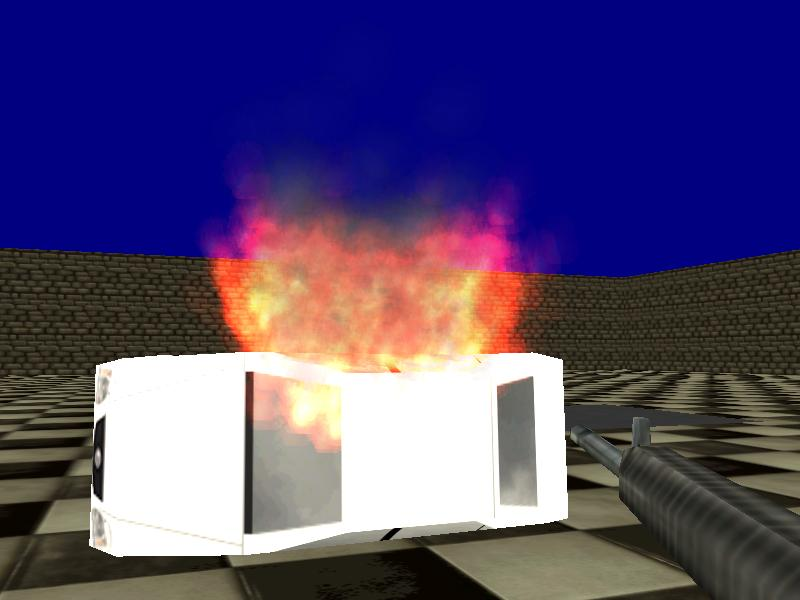Q: I would like to have a flash (smashing like) appear in random places on my model. How do I do that? A: Here's an example that generates a sprite in random areas of a model, every 3 seconds.   function smashed_effect() {        while (my.scale_x < 2)        {                my.scale_x += 1 * time_step;                my.scale_y = my.scale_x;                wait (1);        }        while (my.scale_x > 0.1)                {                my.scale_x -= 3 * time_step;                my.scale_y = my.scale_x;                wait (1);        }        ent_remove (my); }   action smashed_entity() {        VECTOR temp;        var random_vertex;        while (1)        {                random_vertex = integer(random(ent_vertices (my))) + 1;                vec_for_vertex (temp, my, random_vertex);                ent_create("smashed.pcx", temp.x, smashed_effect);                wait (-3);        } }